# CE 3354 Engineering Hydrology Lecture 20 Reservoir Storage

• Slides: 23
Download presentation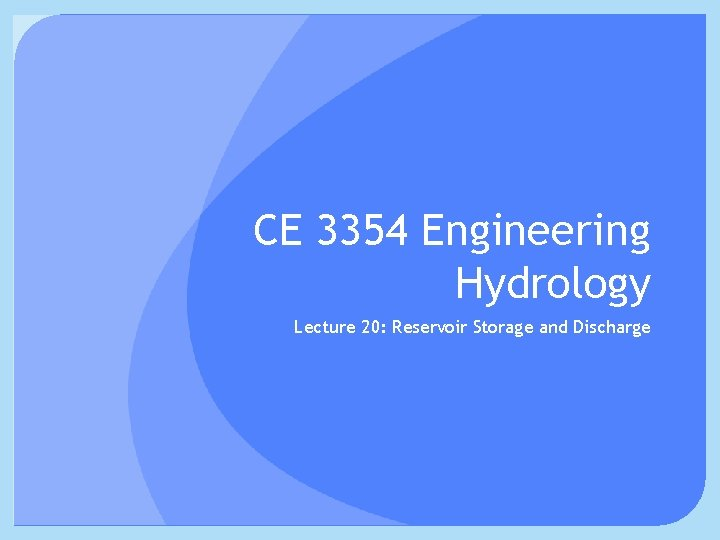CE 3354 Engineering Hydrology Lecture 20: Reservoir Storage and Discharge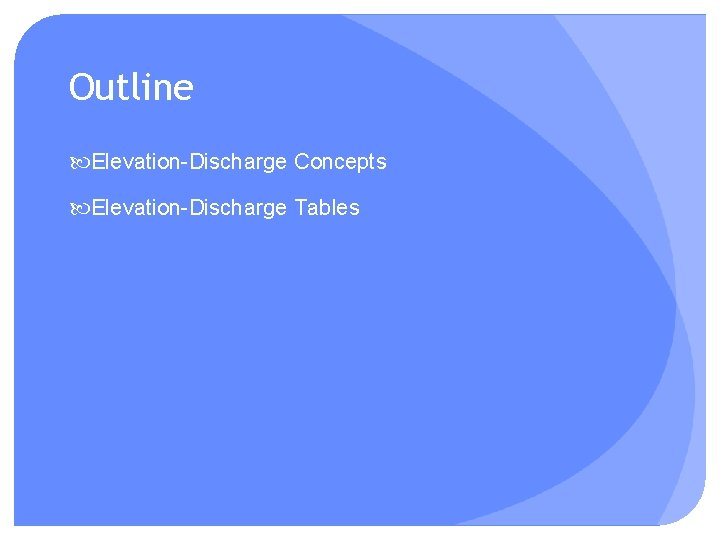Outline Elevation-Discharge Concepts Elevation-Discharge TablesElevation Discharge Elevation. Discharge Determine pool area at different elevations Use hydraulic outlet features to estimate dischargeElevation Discharge Elevation. Discharge Determine pool area at different elevations Use hydraulic outlet features to estimate dischargeElevation Discharge Elevation. Discharge Determine pool area at different elevations Use hydraulic outlet features to estimate dischargeElevation Discharge Elevation. Discharge Determine pool area at different elevations Use hydraulic outlet features to estimate discharge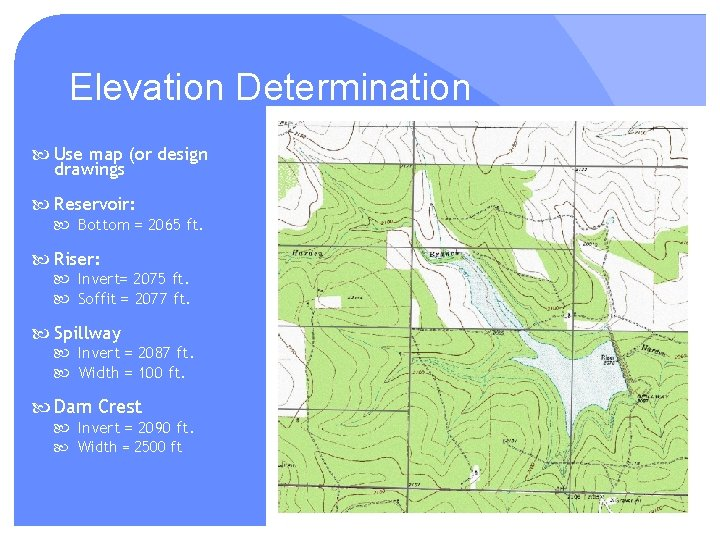Elevation Determination Use map (or design drawings Reservoir: Bottom = 2065 ft. Riser: Invert= 2075 ft. Soffit = 2077 ft. Spillway Invert = 2087 ft. Width = 100 ft. Dam Crest Invert = 2090 ft. Width = 2500 ft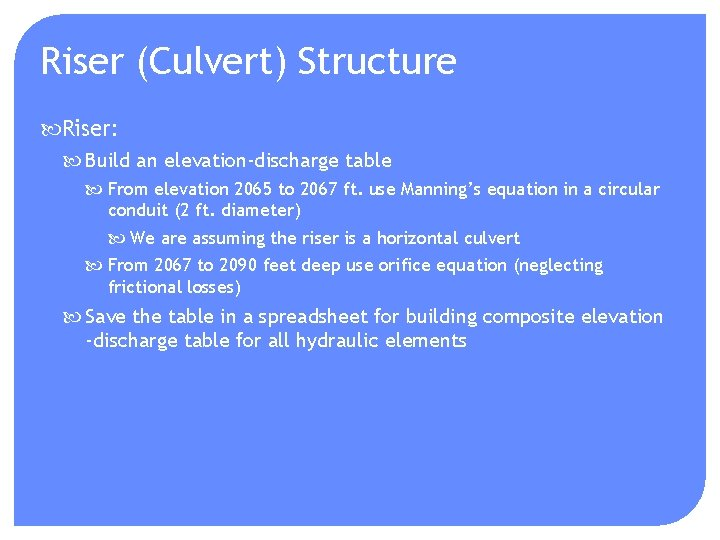Riser (Culvert) Structure Riser: Build an elevation-discharge table From elevation 2065 to 2067 ft. use Manning’s equation in a circular conduit (2 ft. diameter) We are assuming the riser is a horizontal culvert From 2067 to 2090 feet deep use orifice equation (neglecting frictional losses) Save the table in a spreadsheet for building composite elevation -discharge table for all hydraulic elementsRiser (Culvert) Structure Modify Manning’s Calculator CMM pg 162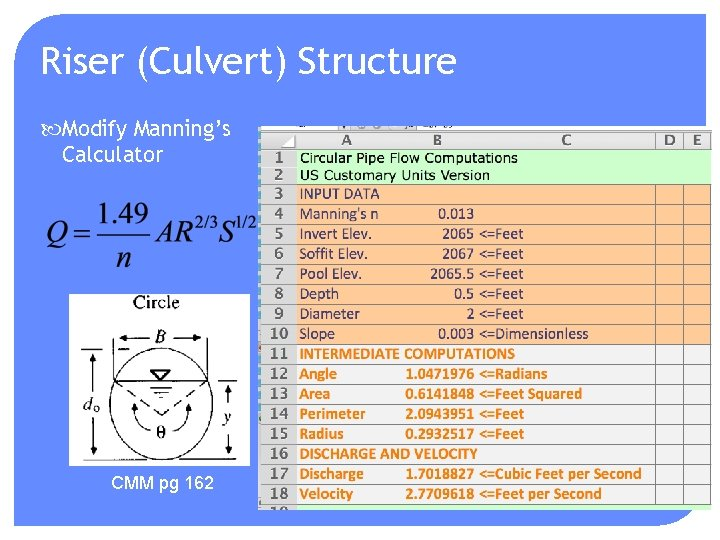Riser (Culvert) Structure Modify Manning’s Calculator CMM pg 162Riser (Culvert) Structure Modify Manning’s Calculator CMM pg 162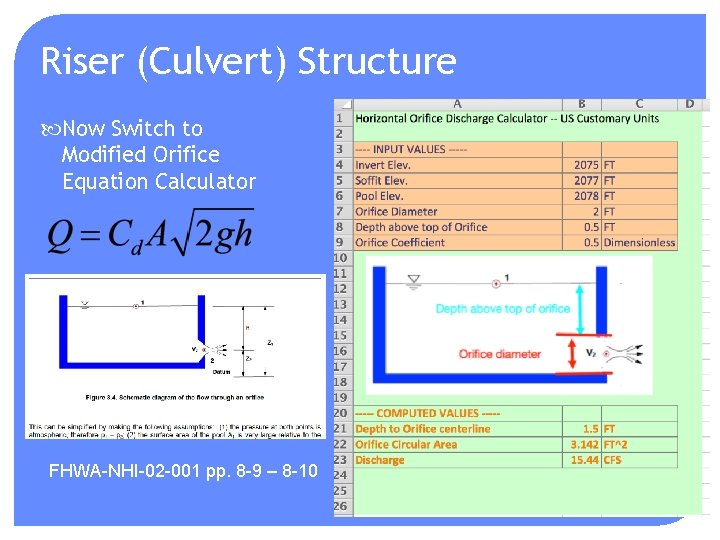Riser (Culvert) Structure Now Switch to Modified Orifice Equation Calculator FHWA-NHI-02 -001 pp. 8 -9 – 8 -10Riser (Culvert) Structure Now Switch to Modified Orifice Equation Calculator FHWA-NHI-02 -001 pp. 8 -9 – 8 -10Spillway Structure Now Add Spillway starting at z = 2087+ ft. Need to select a spillway equation: FHWA-NHI-02 -001 pp. 8 -9 – 8 -10Spillway TypesSpillway Structure Now Add Spillway starting at z = 2087+ ft. Definitions of weir terms – namely approach depth FHWA-NHI-02 -001 pp. 8 -9 – 8 -10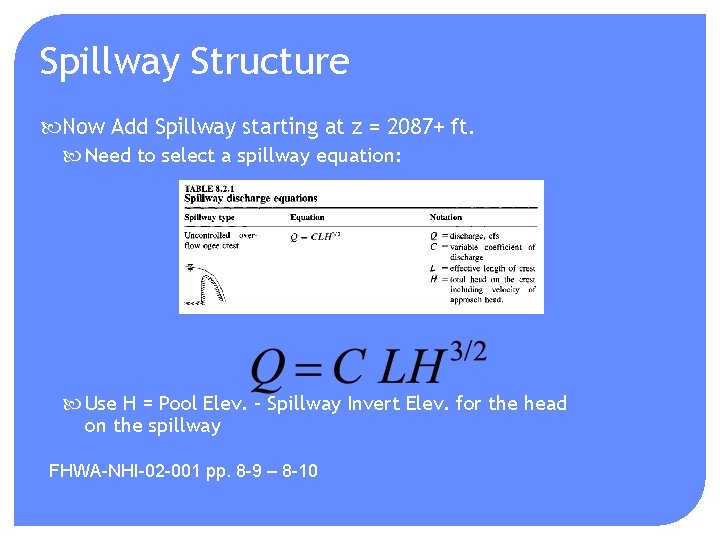Spillway Structure Now Add Spillway starting at z = 2087+ ft. Need to select a spillway equation: Use H = Pool Elev. – Spillway Invert Elev. for the head on the spillway FHWA-NHI-02 -001 pp. 8 -9 – 8 -10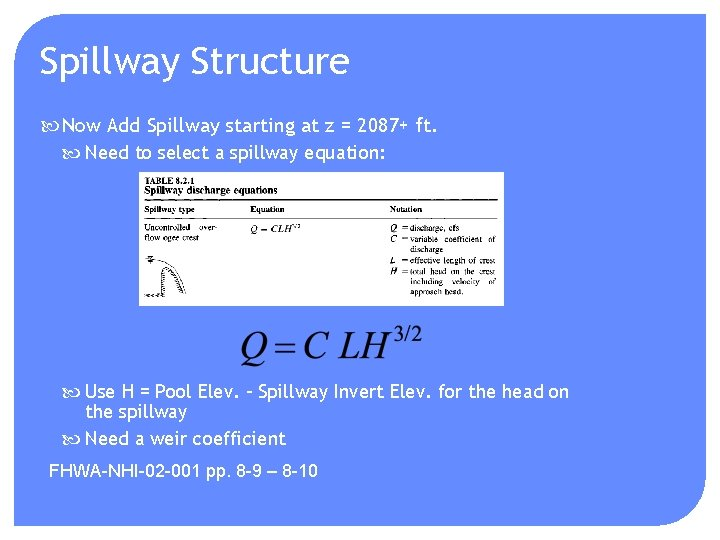Spillway Structure Now Add Spillway starting at z = 2087+ ft. Need to select a spillway equation: Use H = Pool Elev. – Spillway Invert Elev. for the head on the spillway Need a weir coefficient FHWA-NHI-02 -001 pp. 8 -9 – 8 -10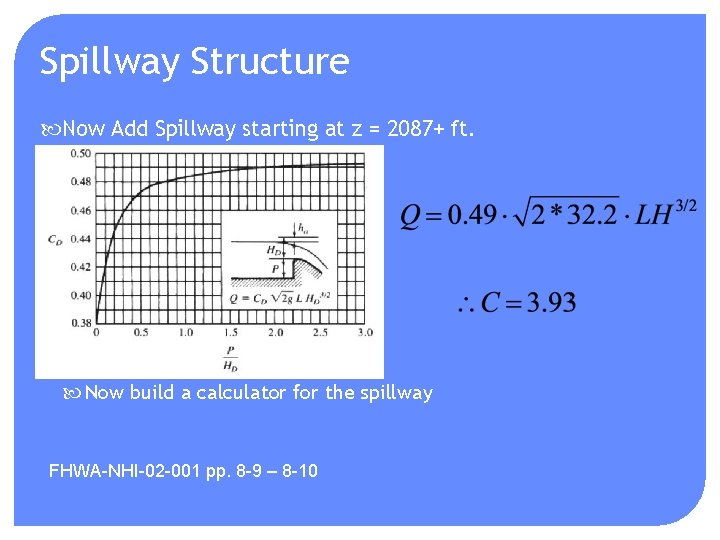Spillway Structure Now Add Spillway starting at z = 2087+ ft. Now build a calculator for the spillway FHWA-NHI-02 -001 pp. 8 -9 – 8 -10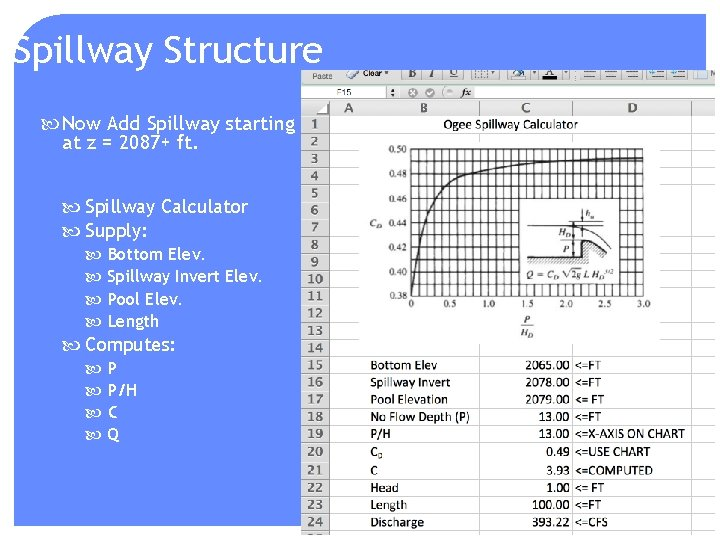Spillway Structure Now Add Spillway starting at z = 2087+ ft. Spillway Calculator Supply: Bottom Elev. Spillway Invert Elev. Pool Elev. Length Computes: P P/H C QSpillway Structure Now Add Spillway starting at z = 2087+ ft. Systematically apply in 0. 5 foot intervals (like all the rest) to build the spillway portion of the table. Use 2500 foot spillway width for the dam crest with a H = 0. 5 feetCombined Structures Elevation-Discharge Table Ready for HEC-HMS or for homebrew level pool routing Use same method for the crossing Multiple culverts (multiply Q by how many culverts Road surface is the spillway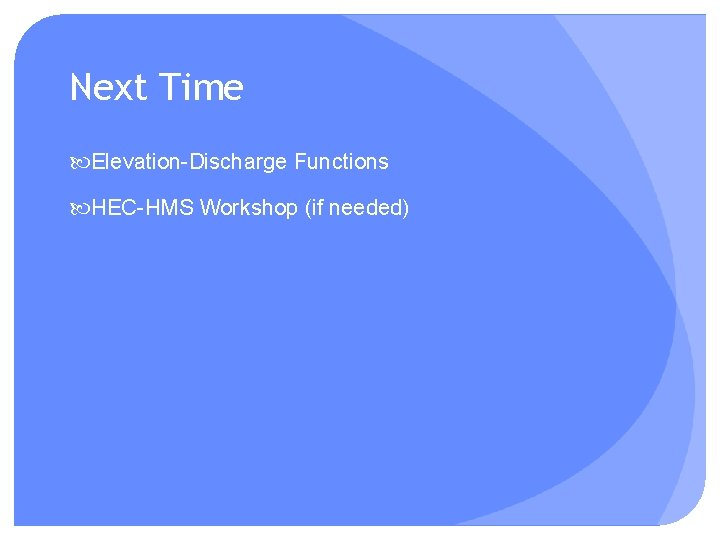Next Time Elevation-Discharge Functions HEC-HMS Workshop (if needed)